# Density Profiles and Contour Plots from Scatter Plots

Page under construction...

Suppose you have a list of points, for example (x,y) pairs. You can easily draw these as a scatter plot, but for a large number of points, some sort of density or contour plot is called for. To do this, we need to generate an estimated density profile... maybe as a sum of gaussian/normal distributions (one for each data point) in order to assign a height (or z-value) over the (x,y) plane.

## Ramachandran Phi/Psi Angles

To explore how we can create a density mapping from a set of datapoints we will need some sample data. For example, top500_psi_phi_bio.tsv can be used to draw Ramachandran Plots. This is about 100,000 pairs of phi/psi proteins backbone torsion angles (ϕ,ψ) extracted from the "Top 500" PDB list using BioPython (see this page).

Disclaimer: I do not consider this angle data to be complete or entirely accurate, for reasons explained on the linked pages.

This is a "Tab Separated Variables" (TSV) file - just plain text with the fields split up using the tab character. So that it can easily be understood by Microsoft Excel, it contains the string "=NA()" where a value is missing. We can load this file in R as follows, filtering out the lines with missing data, and selecting the only the important columns:

```> data = read.table("top500_psi_phi_bio.tsv", header=TRUE, na.strings="=NA()")
> good.data <- data[!is.na(data\$Phi) & !is.na(data\$Psi), c("Phi","Psi","Type")]
> rm(data)
> good.data[28:38,]
Phi        Psi    Type
28  -97.72288  70.769076 Generic
29   70.94610  21.716954     GLY
30  -81.18876 104.080240 Generic
31  -62.00479 130.228887 Generic
32  -94.38121 -50.701498 Generic
33 -161.92154 152.688848 Generic
34  -93.01950  -6.699262 Generic
35  -69.61100 146.683574  PrePRO
36  -70.84696 -10.847430     PRO
37  -84.09883 122.448889 Generic
38  -63.94087 -26.885134 Generic```

If you are familar with Ramachadran Plots, you'll understand what the Type column in the data is all about. We can use it to create four subsets of data:

```> generic <- good.data[good.data\$Type=="Generic",c("Phi","Psi")]
> prepro <- good.data[good.data\$Type=="PrePRO",c("Phi","Psi")]
> proline <- good.data[good.data\$Type=="PRO",c("Phi","Psi")]
> glycine <- good.data[good.data\$Type=="GLY",c("Phi","Psi")]
```

## Scatter Plots

Now we can do a (ϕ,ψ) scatter plot like so - notice how we can get greek letters in the plot captions:

```> plot(good.data[,"Phi"],good.data[,"Psi"],
xlim=c(-180,180), ylim=c(-180,180), cex=0.1, pch=20,
xlab=expression(phi), ylab=expression(psi), main="All residues")```

The option pch=20 gives dots (rather than the default of circles) for each datapoint, and cex=0.1 makes them smaller than by default. This gives the following: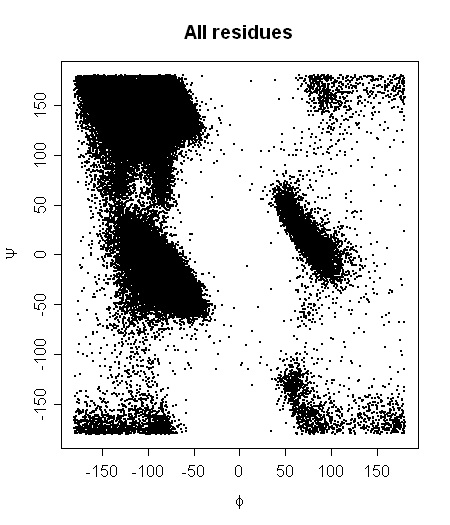We can plot the four data subsets individually in much the same way: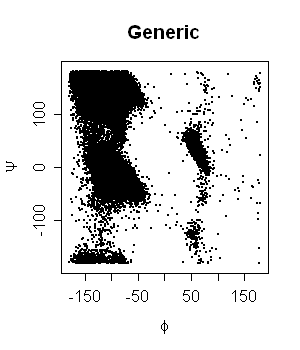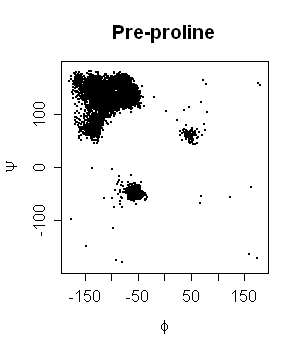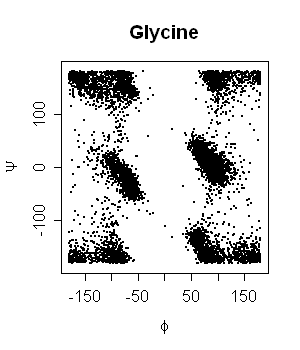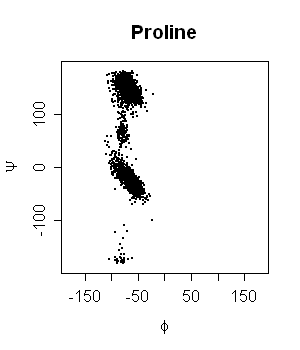Just by eye, its quite clear that my data includes a number of stray points not shown in the equivalent figures from Lovell et al. (2003) (see references).

## Smoothing the data

Now I want to smooth these figures and show them as contour plots. One idea would be to start with a two-dimensional histogram.

My first instinct was to build up a density map by summation of gaussian normals about each (ϕ,ψ) data point, hand tuning the distribution width. I found two R functions, hde2d and sm.density that appear to do this.

In their paper, Lovell et al. (2003) (see references) used a complicated two-step scheme to generate a density profile using cosine functions centred on each data point.

Note that for this example (Ramachadran Plots), our coordinates actually wrap round (-180° = +180°) and this should really be considered when smoothing the data.

## kde2d - Two-Dimensional Kernel Density Estimation

There is an R function called kde2d (Two-Dimensional Kernel Density Estimation), available in the MASS library - part of the VR bundle (Venables & Ripley). The function's description says it is a "Two-dimensional kernel density estimation with an axis-aligned bivariate normal kernel, evaluated on a square grid" - see help(kde2d).

The MASS library was produced for Venables & Ripley's book, Modern Applied Statistics with S, and later ported from the S language to R.

I thought I would give kde2d a go... A note of warning, applying this function to the full generic dataset requires a lot of memory (about 1.3 GB on my Linux machine), so I'm going to stick with the much smaller proline only list for now:

```> library(MASS)
> proline.density <- kde2d(proline\$Phi,proline\$Psi, n=361, lims=c(-180,180,-180,180), h=c(10,10))
> summary(proline.density)
Length Class  Mode
x    360 -none- numeric
y    360 -none- numeric
z 129600 -none- numeric
> sum(proline.density\$z)
 0.9671689
> max(proline.density\$z)
 0.0004633823
> min(proline.density\$z)
 0```

Now, Lovel et al. divided their plots into three regions using 99.8% (allowed) and 98% (favoured) levels (for the glycine, proline and pre-proline plots) or 99.95% (allowed) and 98% (favored) levels for the generic case. So, lets try and do the same (getting the cuttoffs took a little trial and error):

```> proline.total <- sum(proline.density\$z)
> 100*sum(proline.density\$z[proline.density\$z > 0.00000123]) / proline.total
 99.8003
> 100*sum(proline.density\$z[proline.density\$z > 0.00000726]) / proline.total
 97.99556
> filled.contour(proline.density, xlab=expression(phi), ylab=expression(psi),
main="Proline", levels=c(0,0.00000123,0.00000726,1),
xlim=c(-180,180), ylim=c(-180,180), asp=1,
col=c('#FFFFFF','#C0FFC0','#80FF80'))```

I would like to draw this figure without the colour key, but haven't yet worked out how to turn if off, so the following pictures have been cropped.

Anyway, this gives the figure on the left below. If we change the smoothing parameter from h=c(10,10) to c(20,20) and recalculate the contour levels, we get the figure on the right: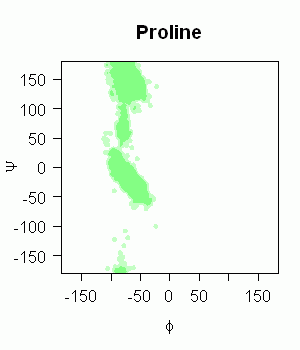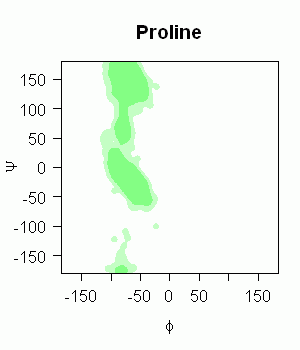This is close to the images by Lovell et al., and is probably just spoilt by the extra stray points in my dataset, as can be seen in this superposition, again using h=c(20,20) in kde2d: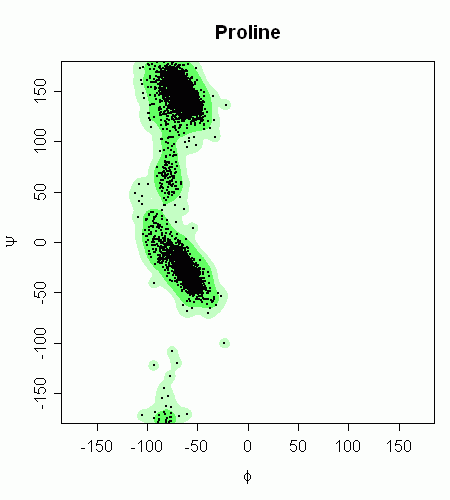## Nonparametric density estimation - R Function sm.density

There is another smoothing function using normal kernals in the sm library:

```> library(sm)
> proline.density <- sm.density(proline, h=c(5,5), nbins=361,
xlim=c(-180,180), ylim=c(-180,180),
xlab="phi", ylab="psi", zlab="")```

That gives this figure: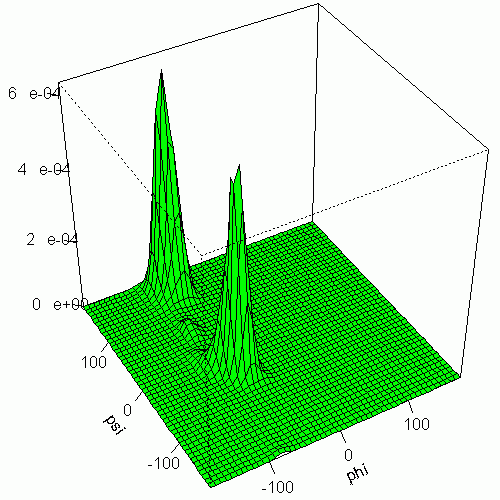We can produce a filled contour plot for this too:

```> proline.total <- sum(proline.density\$estimate)
> 100*sum(proline.density\$estimate[proline.density\$estimate > 0.00000573]) / proline.total
 98.01606
> 100*sum(proline.density\$estimate[proline.density\$estimate > 0.00000068]) / proline.total
 99.80196

> png("proline_sm_h5.png", width=400, height=350)
> filled.contour(proline.density\$estimate, xlab=expression(phi), ylab=expression(psi),
main="Proline",
x = proline.density\$eval.points[,"xgrid"],
y = proline.density\$eval.points[,"ygrid"],
xlim=c(-180,180), ylim=c(-180,180), asp=1,
levels=c(0,0.00000068,0.00000573,1),
col=c('#FFFFFF','#C0FFC0','#80FF80'))
> dev.off()```

Cropping of the colour key again, this gives: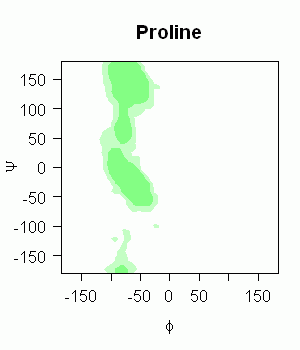## Remarks

The figures produced from hde2d and sm.density are broadly the same. However their numerical values differ - probably because only hde2d attempts to normalise its values to give a sum of one.